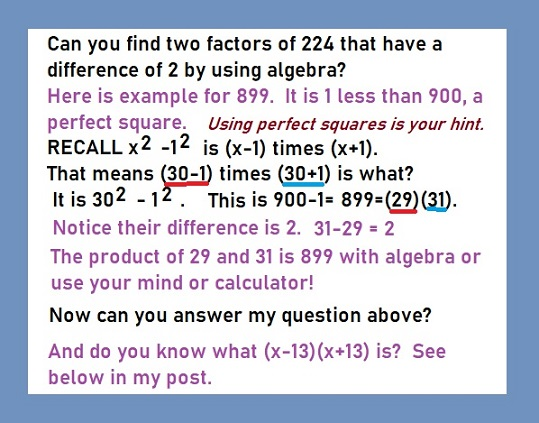# Introductory math for “Lockdown math”

Posted on: April 20, 2020 Posted by: Susan Johnsey Comments: 0If you are interested in the Youtube channel 3blue1brown then you may have watched their first episode for Lockdown Math that was streamed April 17, 2020. He presented several topics of math pertaining to quadratics and gave the example of one of his followers using it for video games. I thought I would help with at least 2 of the topics by giving more explanation of the math he was using and a few problems to test your abilities. The first topic is sum and difference of two numbers (or variables) Here goes!

Let us begin by reviewing the numbers called “perfect squares”. WRITE these down. We will be needing them soon. I begin with 1 squared then 2 squared and then 3 squared and so on. 1, 4, 9, 16, 25, 36, 49, 64, 81, 100, 121, 144, 169, 196, 225, 256 (we just did 16 squared ). Now also know 20 squared is 400, 25 squared is 625 and 60 squared is 3600. I jumped to 3600 because in the video they found factors (numbers you multiply) to get 1 less than 3600. Do you recall that question. What are the factors of 3599?

We will get back to those in a few minutes. Now let’s do algebra. Multiply (x-3)(x+3) Many students learned this by using FOIL. Multiply First terms: x times x is x^2. ( ^2 means exponent of 2, or squared). Multiply Outside terms: x times (+3) = +3x. Multiply Inside terms -3 times x is -3x. Multiply Last terms: -3 tmes +3 is -9. NOW add these 4 terms together: x^2 +3x -3x -9. Simplify to x^2 – 9. You do know the 3x-3x or also written -3x+3x = 0. We have (x-3)(x+3) = x^2 – 9.

• NOW you do these using FOIL: (x-4)(x+4)=____________________________
• (x-5)(x+5) =____________________________________________________________
• (x-1)(x+1) = ___________________________________________________________
• Can you do this one without all the steps? did you see the pattern?(x-13)(x+13) =____________________________________YOu need your perfect squares that you wrote down.
• Now do this one using the same method (that is, do NOT do the math work inside the 2 parentheses). Use FOIL to multiply: (60-1)(60+1) Did you get 60^2 -1^2? Now simplify the 60^2 -1^2 = 3600 -1 = 3599. Thus we know two factors to multiply to get the 3599. The man at the hotel answered 59 times 61, Our 60-1 and 60+1. Use this method to find two factors for 624 and Email me your work and answer!! Hint look at the list of perfect squares. Which is 1 more than this number?

Answers for the 4 problems above are x^2 – 16, x^2 – 25, x^2 -1, x^2 -169.

Multiply these with FOIL Send the answers if you want me to check them. (x-3)(x+4) and (2x-7)(x+1) If these are too hard ask questions and try them any way.

Do you want to know more about Quadratics? Look in my Topics box on the right side. If you would like some problems to try let me know and include how much you know about algebra so I can make them hard or easy! Use the CONTACT page.